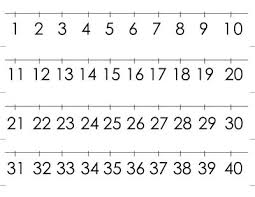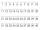# How many 4

How many four-digit numbers that are divisible by ten can be created from the numbers 3, 5, 7, 8, 9, 0 such no digits repeats?

n =  60

### Step-by-step explanation:Did you find an error or inaccuracy? Feel free to write us. Thank you!Tips to related online calculators
Would you like to compute count of combinations?

## Related math problems and questions:

• Divisible by fiveHow many different three-digit numbers divisible by five can we create from the digits 2, 4, 5? We can repeat the digits in the created number.
• Three digits numberHow many are three-digit integers such that they no digit repeats?
• Three-digit numbersHow many are all three-digit numbers that are made up of digits 0,2,5,7 and are divisible by 9 if the digits can be repeated?
• Three digits numberFrom the numbers 1, 2, 3, 4, 5 create three-digit numbers that digits not repeat and number is divisible by 2. How many numbers are there?
• Dd 2-digit numbersFind all odd 2-digit natural numbers compiled from digits 1; 3; 4; 6; 8 if the digits are not repeated.
• Three-digit integersHow many three-digit natural numbers exist that do not contain zero and are divisible by five?
• Twenty-fiveHow many are three-digit natural numbers divisible by 25?
• DigitsHow many odd four-digit numbers can we create from digits: 0, 3, 5, 6, 7? (a) the figures may be repeated (b) the digits may not be repeated
• Five-digitFind all five-digit numbers that can be created from numbers 12345 so that the numbers are not repeated and then numbers with repeated digits. Give the calculation.
• SymmetryEva loves symmetry in shapes and numbers. Yesterday she invented a completely new kind of symmetry - divisible symmetry. She wrote all five-digit numbers with different digits with the following property: the first digit is divisible by 1, the second by 2
• Five-digit numberAnna thinks of a five-digit number that is not divisible by three or four. If he increments each digit by one, it gets a five-digit number that is divisible by three. If he reduces each digit by one, he gets a five-digit number divisible by four. If it sw
• Inverted nineIn the hotel Inverted Nine, each hotel room number is divisible by 6. How many rooms can we count with the three-digit number registered by digits 1,8,7,4,9?
• How manyHow many double-digit numbers greater than 30 we can create from digits 0, 1, 2, 3, 4, 5? Numbers cannot be repeated in a two-digit number.
• DigitsHow many five-digit numbers can be written from numbers 0.3,4, 5, 7 that is divided by 10, and if digits can be repeated?
• What are 3What are the two digits which when inserted in the blank spaces will make 234 _ _ divisible by 8?
• Three-digit numbersWe have digits 0,1,4,7 that cannot be repeated. How many three-digit numbers can we write from them? You can help by listing all the numbers.
• CombinationsHow many different combinations of two-digit number divisible by 4 arises from the digits 3, 5 and 7?